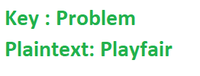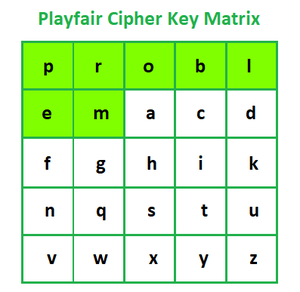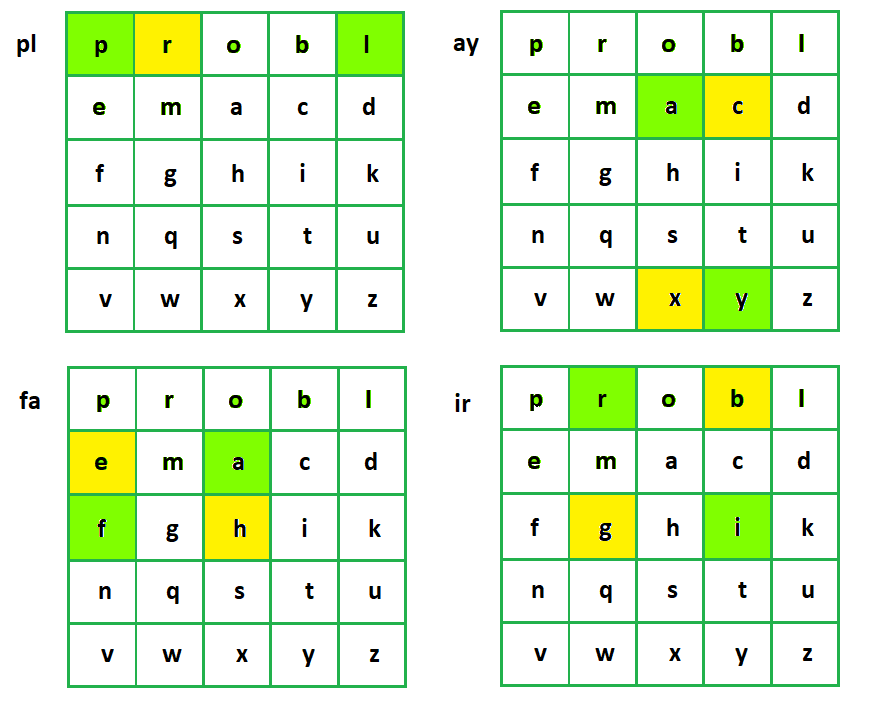GFG App
Open AppBrowser
Continue

# Java Program to Encode a Message Using Playfair Cipher

The Playfair cipher is one of the traditional ciphers which comes under the category of substitution ciphers. In Playfair Cipher, unlike traditional cipher, we encrypt a pair of alphabets (digraphs) instead of a single alphabet. In Playfair cipher, initially, a key table is created. The key table is a 5×5 matrix consisting of alphabets that acts as the key for encryption of the plaintext. Each of the 25 alphabets must be unique and one letter of the alphabet (usually ‘j’) is omitted from the table, as we need only 25 alphabets instead of 26. If the plaintext contains ‘j’, then it is replaced by ‘i’.

Process for Playfair Cipher:

1. The plaintext message is split into pairs of two letters (digraphs). If the plaintext has an odd number of characters, append ‘z’ to the end to make the message of even length.
2. Identify any double letters placed side by side in the plaintext and replace the second occurrence with an ‘x’ e.g. ‘hello’ -> ‘he lx lo’.
3. Now, locate the letters in the 5×5 key table.
4. Use the following rules for encryption of plaintext:
• If the letters appear on the same row of your table, replace them with the letters to their immediate right respectively (wrapping around to the left side of the row if a letter in the original pair was on the right side of the row).
• If the letters appear on the same column of your table, replace them with the letters immediately below respectively (wrapping around to the top side of the column if a letter in the original pair was on the bottom side of the column)
• If the letters are not on the same row or column, replace them with the letters in their own row but in the same column as the other letter.

The decryption process of Playfair cipher is the same encryption process, but it is applied in a reverse manner. The receiver has the same key and can create the same key table, and he uses it to decrypt the ciphertext messages generated using that key.

Example:Inputs for playfair cipherPlayfair Cipher Key MatrixCiphertext generation using Playfair Cipher

## Java

 `// Java Program to Encode a Message Using Playfair Cipher`   `import` `java.io.*;` `import` `java.util.*;`   `class` `Playfair {` `    ``String key;` `    ``String plainText;` `    ``char``[][] matrix = ``new` `char``[``5``][``5``];`   `    ``public` `Playfair(String key, String plainText)` `    ``{` `        ``// convert all the characters to lowercase` `        ``this``.key = key.toLowerCase();` `      `  `        ``this``.plainText = plainText.toLowerCase();` `    ``}`   `    ``// function to remove duplicate characters from the key` `    ``public` `void` `cleanPlayFairKey()` `    ``{` `        ``LinkedHashSet set` `            ``= ``new` `LinkedHashSet();` `      `  `        ``String newKey = ``""``;` `      `  `        ``for` `(``int` `i = ``0``; i < key.length(); i++)` `            ``set.add(key.charAt(i));`   `        ``Iterator it = set.iterator();` `      `  `        ``while` `(it.hasNext())` `            ``newKey += (Character)it.next();`   `        ``key = newKey;` `    ``}`   `    ``// function to generate playfair cipher key table` `    ``public` `void` `generateCipherKey()` `    ``{` `        ``Set set = ``new` `HashSet();` `      `  `        ``for` `(``int` `i = ``0``; i < key.length(); i++)` `        ``{` `            ``if` `(key.charAt(i) == ``'j'``)` `                ``continue``;` `            ``set.add(key.charAt(i));` `        ``}`   `        ``// remove repeated characters from the cipher key` `        ``String tempKey = ``new` `String(key);` `      `  `        ``for` `(``int` `i = ``0``; i < ``26``; i++) ` `        ``{` `            ``char` `ch = (``char``)(i + ``97``);` `            ``if` `(ch == ``'j'``)` `                ``continue``;` `          `  `            ``if` `(!set.contains(ch))` `                ``tempKey += ch;` `        ``}`   `        ``// create cipher key table` `        ``for` `(``int` `i = ``0``, idx = ``0``; i < ``5``; i++)` `            ``for` `(``int` `j = ``0``; j < ``5``; j++)` `                ``matrix[i][j] = tempKey.charAt(idx++);`   `        ``System.out.println(``"Playfair Cipher Key Matrix:"``);` `      `  `        ``for` `(``int` `i = ``0``; i < ``5``; i++)` `            ``System.out.println(Arrays.toString(matrix[i]));` `    ``}`   `    ``// function to preprocess plaintext` `    ``public` `String formatPlainText()` `    ``{` `        ``String message = ``""``;` `        ``int` `len = plainText.length();` `      `  `        ``for` `(``int` `i = ``0``; i < len; i++)` `        ``{` `            ``// if plaintext contains the character 'j',` `            ``// replace it with 'i'` `            ``if` `(plainText.charAt(i) == ``'j'``)` `                ``message += ``'i'``;` `            ``else` `                ``message += plainText.charAt(i);` `        ``}`   `        ``// if two consecutive characters are same, then` `        ``// insert character 'x' in between them` `        ``for` `(``int` `i = ``0``; i < message.length(); i += ``2``) ` `        ``{` `            ``if` `(message.charAt(i) == message.charAt(i + ``1``))` `                ``message = message.substring(``0``, i + ``1``) + ``'x'` `                          ``+ message.substring(i + ``1``);` `        ``}` `      `  `        ``// make the plaintext of even length` `        ``if` `(len % ``2` `== ``1``)` `            ``message += ``'x'``; ``// dummy character` `      `  `        ``return` `message;` `    ``}`   `    ``// function to group every two characters` `    ``public` `String[] formPairs(String message)` `    ``{` `        ``int` `len = message.length();` `        ``String[] pairs = ``new` `String[len / ``2``];` `      `  `        ``for` `(``int` `i = ``0``, cnt = ``0``; i < len / ``2``; i++)` `            ``pairs[i] = message.substring(cnt, cnt += ``2``);` `      `  `        ``return` `pairs;` `    ``}`   `    ``// function to get position of character in key table` `    ``public` `int``[] getCharPos(``char` `ch)` `    ``{` `        ``int``[] keyPos = ``new` `int``[``2``];` `      `  `        ``for` `(``int` `i = ``0``; i < ``5``; i++) ` `        ``{` `            ``for` `(``int` `j = ``0``; j < ``5``; j++)` `            ``{` `              `  `                ``if` `(matrix[i][j] == ch)` `                ``{` `                    ``keyPos[``0``] = i;` `                    ``keyPos[``1``] = j;` `                    ``break``;` `                ``}` `            ``}` `        ``}` `        ``return` `keyPos;` `    ``}`   `    ``public` `String encryptMessage()` `    ``{` `        ``String message = formatPlainText();` `        ``String[] msgPairs = formPairs(message);` `        ``String encText = ``""``;` `      `  `        ``for` `(``int` `i = ``0``; i < msgPairs.length; i++) ` `        ``{` `            ``char` `ch1 = msgPairs[i].charAt(``0``);` `            ``char` `ch2 = msgPairs[i].charAt(``1``);` `            ``int``[] ch1Pos = getCharPos(ch1);` `            ``int``[] ch2Pos = getCharPos(ch2);`   `            ``// if both the characters are in the same row` `            ``if` `(ch1Pos[``0``] == ch2Pos[``0``]) {` `                ``ch1Pos[``1``] = (ch1Pos[``1``] + ``1``) % ``5``;` `                ``ch2Pos[``1``] = (ch2Pos[``1``] + ``1``) % ``5``;` `            ``}` `          `  `            ``// if both the characters are in the same column` `            ``else` `if` `(ch1Pos[``1``] == ch2Pos[``1``])` `            ``{` `                ``ch1Pos[``0``] = (ch1Pos[``0``] + ``1``) % ``5``;` `                ``ch2Pos[``0``] = (ch2Pos[``0``] + ``1``) % ``5``;` `            ``}` `          `  `            ``// if both the characters are in different rows` `            ``// and columns` `            ``else` `{` `                ``int` `temp = ch1Pos[``1``];` `                ``ch1Pos[``1``] = ch2Pos[``1``];` `                ``ch2Pos[``1``] = temp;` `            ``}` `          `  `            ``// get the corresponding cipher characters from` `            ``// the key matrix` `            ``encText = encText + matrix[ch1Pos[``0``]][ch1Pos[``1``]]` `                      ``+ matrix[ch2Pos[``0``]][ch2Pos[``1``]];` `        ``}` `      `  `        ``return` `encText;` `    ``}` `}`   `public` `class` `GFG {` `    ``public` `static` `void` `main(String[] args)` `    ``{` `        ``System.out.println(``"Example-1\n"``);` `      `  `        ``String key1 = ``"Problem"``;` `        ``String plainText1 = ``"Playfair"``;` `      `  `        ``System.out.println(``"Key: "` `+ key1);` `        ``System.out.println(``"PlainText: "` `+ plainText1);` `      `  `        ``Playfair pfc1 = ``new` `Playfair(key1, plainText1);` `        ``pfc1.cleanPlayFairKey();` `        ``pfc1.generateCipherKey();` `      `  `        ``String encText1 = pfc1.encryptMessage();` `        ``System.out.println(``"Cipher Text is: "` `+ encText1);`   `        ``System.out.println(``"\nExample-2\n"``);` `      `  `        ``String key2 = ``"Problem"``;` `        ``String plainText2 = ``"Hello"``;` `      `  `        ``System.out.println(``"Key: "` `+ key2);` `        ``System.out.println(``"PlainText: "` `+ plainText2);` `      `  `        ``Playfair pfc2 = ``new` `Playfair(key2, plainText2);` `        ``pfc2.cleanPlayFairKey();` `        ``pfc2.generateCipherKey();` `      `  `        ``String encText2 = pfc2.encryptMessage();` `        ``System.out.println(``"Cipher Text is: "` `+ encText2);` `    ``}` `}`

Output

```Example-1

Key: Problem
PlainText: Playfair
Playfair Cipher Key Matrix:
[p, r, o, b, l]
[e, m, a, c, d]
[f, g, h, i, k]
[n, q, s, t, u]
[v, w, x, y, z]
Cipher Text is: rpcxhegb

Example-2

Key: Problem
PlainText: Hello
Playfair Cipher Key Matrix:
[p, r, o, b, l]
[e, m, a, c, d]
[f, g, h, i, k]
[n, q, s, t, u]
[v, w, x, y, z]
Cipher Text is: faozpb```

My Personal Notes arrow_drop_up